# Equivalent Fractions Examples

Equivalent Fractions Examples, deals with various concepts which are as under:-

• Find Equivalent Fraction With Given Numerator
• Find Equivalent Fraction With Given Denominator

Two or more Fractions are said to be Equivalent Fractions If they represent the same amount or same Fraction.

To get a fraction equivalent to a given fraction, we multiply or divide the numerator and the denominator of the given fraction by the same number (except 0 or 1)

## Equivalent Fractions Examples

Equivalent Fractions Examples – Example 1

Find the four fractions equivalent to 3/2 ?

Solution

To get a fraction equivalent to a given fraction, we multiply or divide the numerator and the denominator of the given fraction by the same number (except 0 or 1)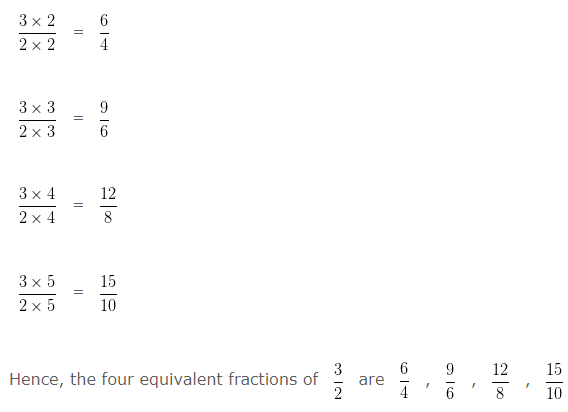Equivalent Fractions Examples – Example 2

Find the four fractions equivalent to 4/3 ?

Solution

To get a fraction equivalent to a given fraction, we multiply or divide the numerator and the denominator of the given fraction by the same number (except 0 or 1)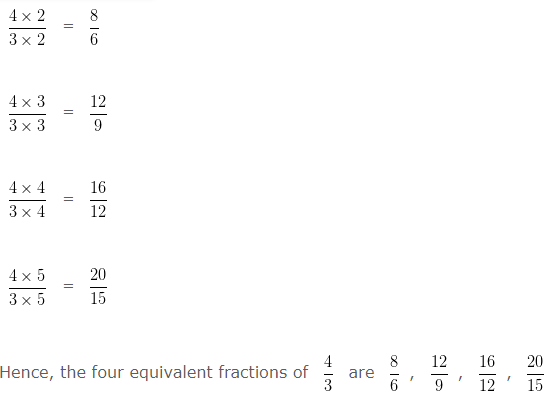### Find Equivalent Fraction With Given Numerator

Equivalent Fractions Examples – Example 3

Write an Equivalent Fraction of 3/5 with Numerator 15 .

Solution

Let,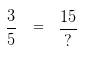In order to obtain an equivalent fraction, we need to multiply the Numerator and Denominator of a given number , by same digit
To get 15 as Numerator, we have to multiply 3 by 5

In order to get an Equivalent Fraction, we have to multiply the Denominator by the same number, i.e, 5
i.e,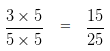Hence, 15/25 is an Equivalent Fraction of 3/5 with Numerator 15 .

Equivalent Fractions Examples – Example 4

Write an Equivalent Fraction of 7/9 with Numerator 21 .

Solution

Let,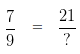In order to obtain an Equivalent fraction, we need to multiply the Numerator and Denominator of a given number , by same digit
To get 21 as Numerator, we have to multiply 7 by 3

In order to get an Equivalent Fraction, we have to multiply the Denominator by the same number, i.e, 3
i.e,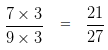Hence, 21/27 is an Equivalent Fraction of 7/9 with Numerator 21.

### Find Equivalent Fraction With Given Denominator

Equivalent Fractions Examples – Example 5

Write an Equivalent Fraction of 20/24 with Denominator 6.

Solution

Let,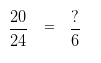In order to obtain an Equivalent fraction, we need to divide the Numerator and Denominator of a given number , by same digit

To get 6 in the Denominator, we need to divide 24 by 4

So, in order to get an Equivalent Fraction, we have to divide the Numerator also by 4

i.e,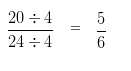Hence, 5/6 is an Equivalent Fraction of 20/24 with Denominator 6

Equivalent Fractions Examples – Example 6

Write an Equivalent Fraction of 15/12 with Denominator 4 .

Solution

Let,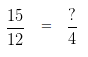In order to obtain an Equivalent fraction, we need to divide the Numerator and Denominator of a given number , by same digit

To get 4 in the Denominator, we need to divide 12 by 3

So, in order to get an Equivalent Fraction, we have to divide the Numerator also by 3

i.e,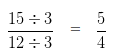Hence, 5/4 is an Equivalent Fraction of 15/12 with Denominator 4